Next: Calculus of Variations Up: Ellipsoidal Potential Theory Previous: Analysis

# Exercises

1. Demonstrate that the volume of an ellipsoid whose bounding surface satisfiesis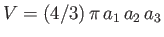.

2. Demonstrate that the moments of inertia about the three Cartesian axes of a homogeneous ellipsoidal body of mass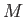, whose bounding surface satisfies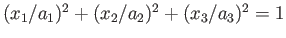, are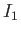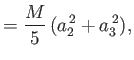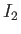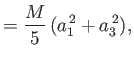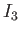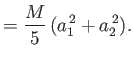3. According to MacCullagh's formula (Fitzpatrick 2012), the gravitational potential a relatively long way from a body of masswhose center of mass coincides with the origin, and whose principal moments of inertial are,, and(assuming that the principal axes coincide with the Cartesian axes), takes the form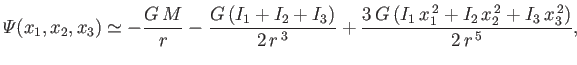where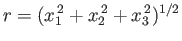. Demonstrate that if the body in question is a homogeneous ellipsoid whose bounding surface satisfiesthen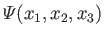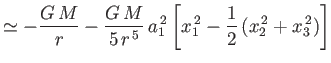(D.37)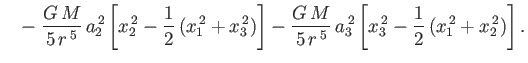4. Show that the gravitational potential external to a homogeneous ellipsoidal body of mass, whose outer boundary satisfies, takes the form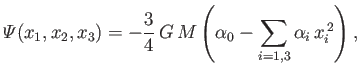(D.38)

where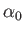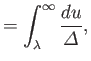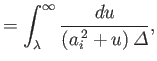and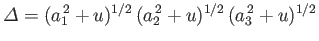. Here,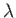is the positive root of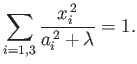Demonstrate that, at large, Equation (D.38) reduces to Equation (D.37).Next: Calculus of Variations Up: Ellipsoidal Potential Theory Previous: Analysis
Richard Fitzpatrick 2016-03-31